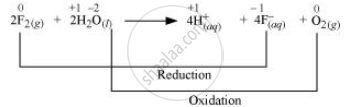Share

# Consider the Reaction of Water with F2 and Suggest, in Terms of Oxidation and Reduction, Which Species Are Oxidized/Reduced. - Chemistry

ConceptWater Chemical Properties of Water

#### Question

Consider the reaction of water with F2 and suggest, in terms of oxidation and reduction, which species are oxidized/reduced.

#### Solution 1

The reaction between fluorine and water can be represented as:

2F_(2(g)) + 2H__2O_(l) -> 4H_((aq))^+ + 4F_((aq))^- + O_(2(g))

This is an example of a redox reaction as water is getting oxidized to oxygen, while fluorine is being reduced to fluoride ion.

The oxidation numbers of various species can be represented as:Fluorine is reduced from zero to (– 1) oxidation state. A decrease in oxidation state indicates the reduction of fluorine.

Water is oxidized from (– 2) to zero oxidation state. An increase in oxidation state indicates oxidation of water.

#### Solution 2

2F2(ag) + 2H20(l)—————> O2(g) + 4H+(aq) + 4F(aq)

In this reaction water acts as a reducing agent and itself gets oxidised to O2 while F2 acts as an oxidising agent and hence itself reduced to F ions.

Is there an error in this question or solution?

#### APPEARS IN

Solution Consider the Reaction of Water with F2 and Suggest, in Terms of Oxidation and Reduction, Which Species Are Oxidized/Reduced. Concept: Water - Chemical Properties of Water.
S